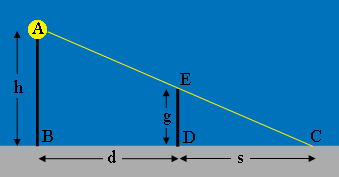SEARCH HOMEMath Central Quandaries & QueriesQuestion from vijay, a student: A girl of height 80 cm is running away from the base of a lamp-post at a speed of 2 m/s. If the lamp is 4 m above the ground, find the length of her shadow after 5 seconds.Hi Vijay,

I would use meters for all the lengths measurements so express the girl's height in meters.

Here is my diagram, not to scale. $A$ is the lamp, $h$ is the lamp height in meters, $g$ is the girl's height in meters and $s$ is the length of her shadow in meters.I assume the girls starts running at $B,$ the base of the lamp. She runs at 2 meters per second for 5 seconds and ends at $D.$ What is $d,$ the distance from $B$ to $D?$

Triangles $ABC$ and $EDC$ are similar. Can you complete the problem now?

Penny* Registered trade mark of Imperial Oil Limited. Used under license.Math Central is supported by the University of Regina and the Imperial Oil Foundation.